# (1 point) For each of the following vector fields F, decide whether it is conservative or...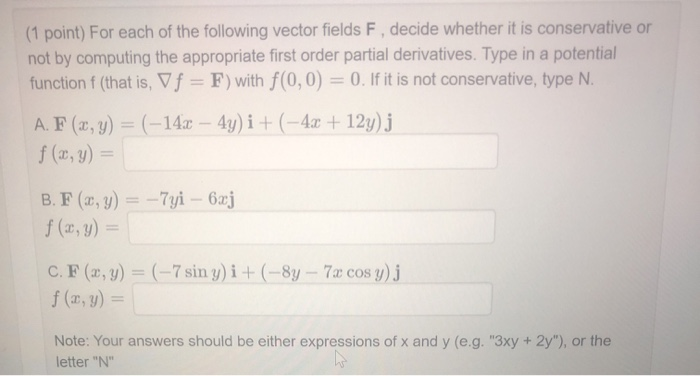(1 point) For each of the following vector fields F, decide whether it is conservative or not by computing the appropriate first order partial derivatives. Type in a potential function f (that is, V f = F) with f(0,0) = 0. If it is not conservative, type N. A. F (x, y) = (-140 – 4y) i + (-4x + 12y)j f (x, y) = B. F (x, y) = -7yi - 6xj f(x,y) = C. F (2, y) = (-7 sin y)i + (-8y - 7x cos y)j f (x, y) = Note: Your answers should be either expressions of x and y (e.g. "3xy + 2y"), or the letter "N"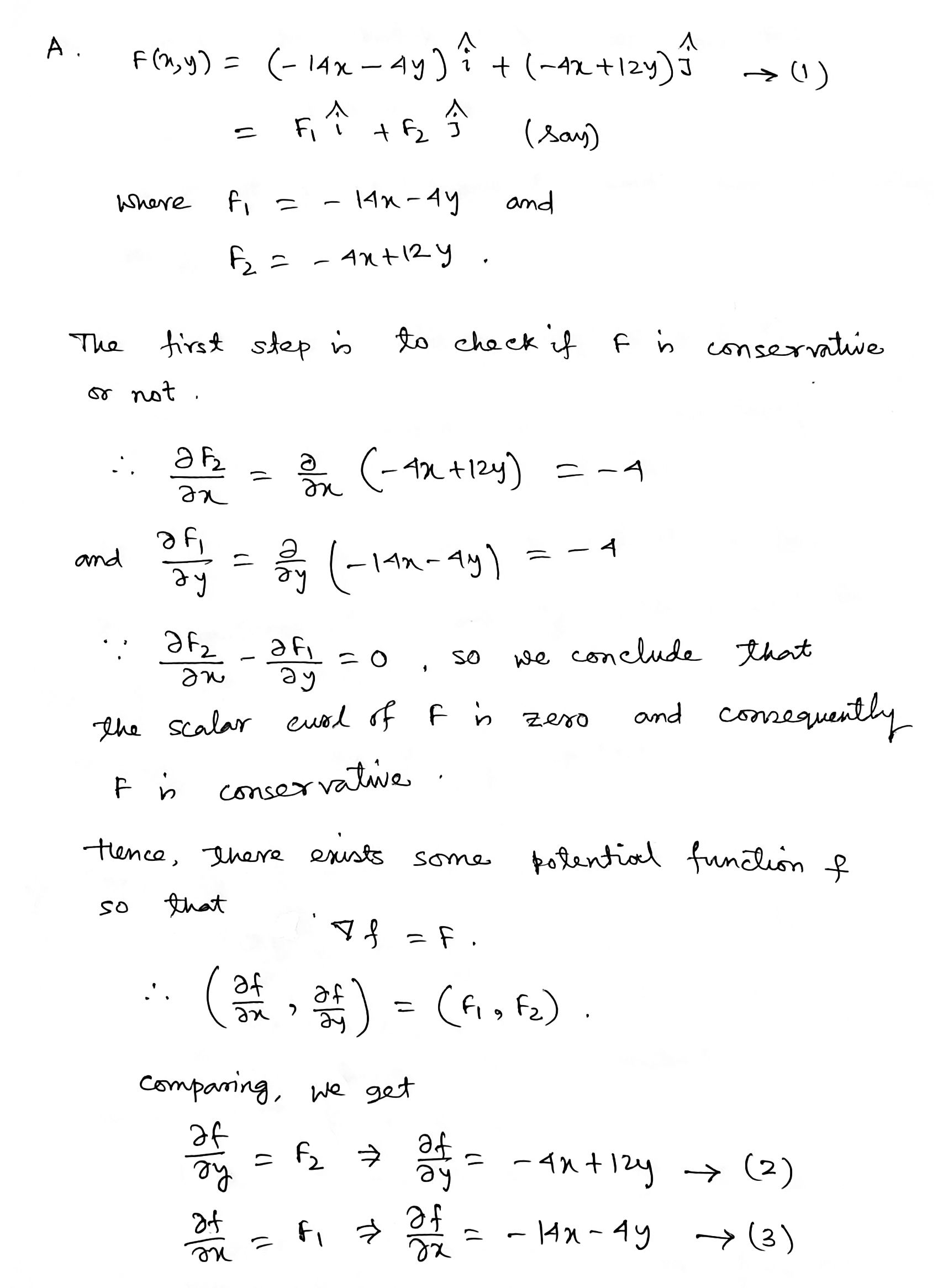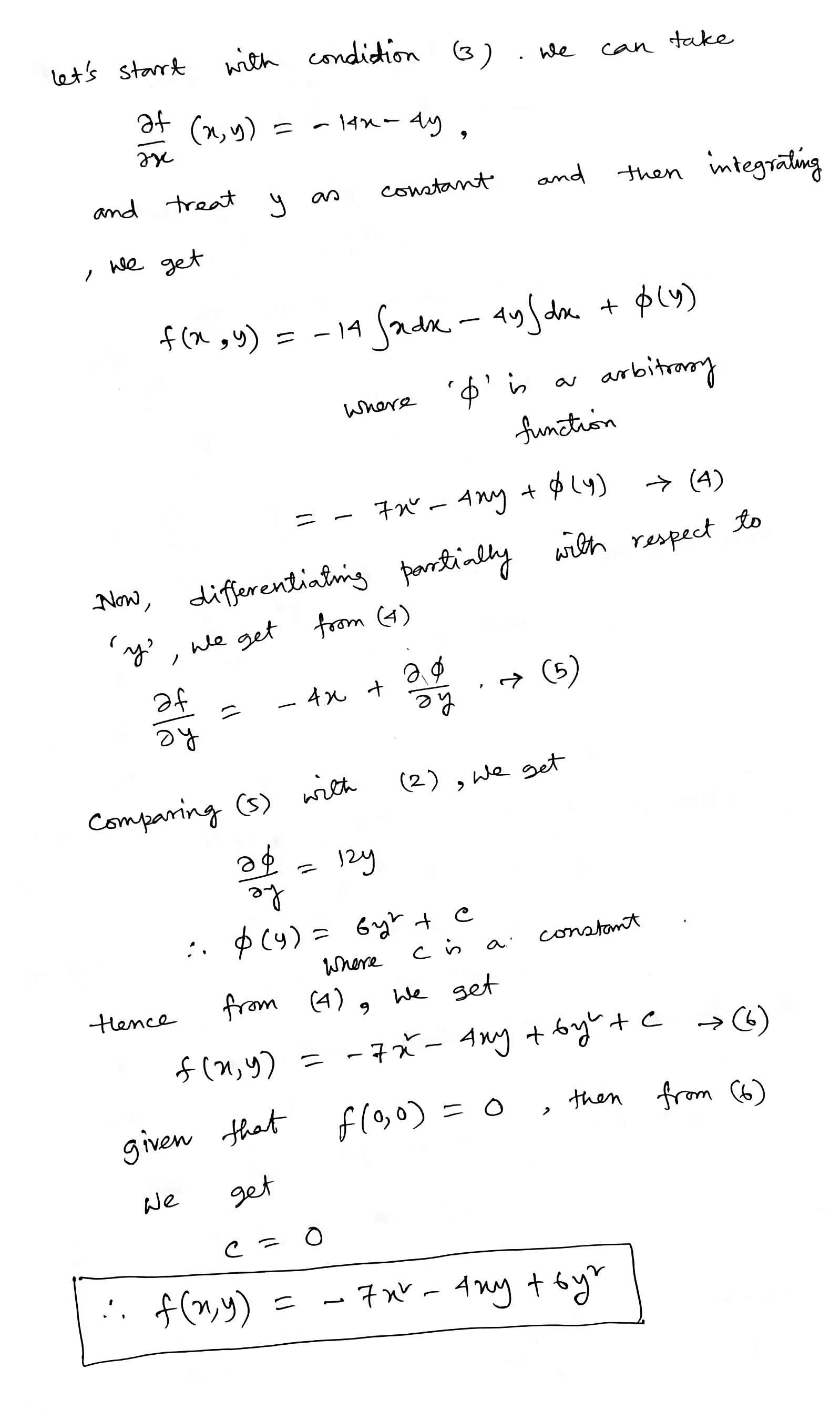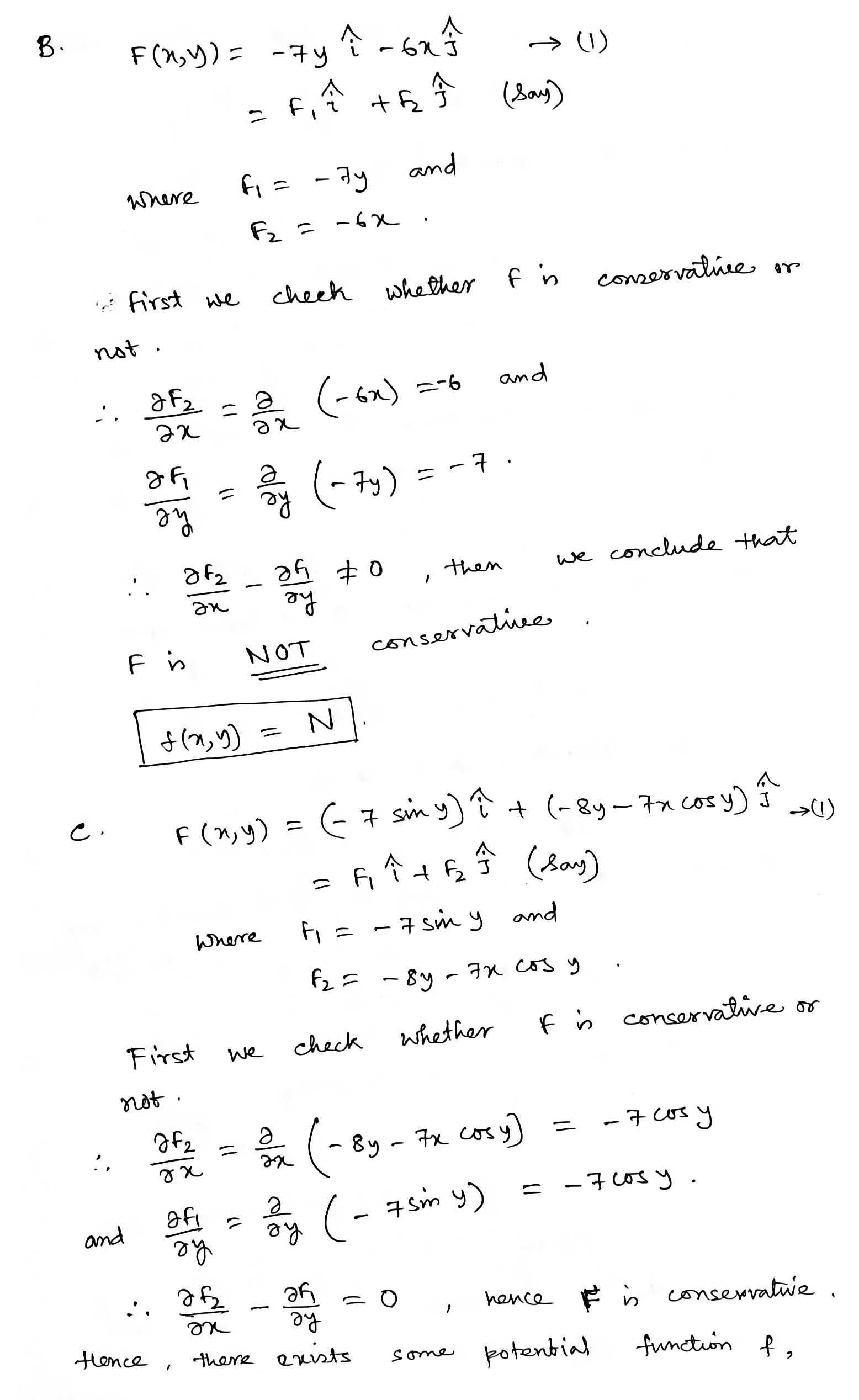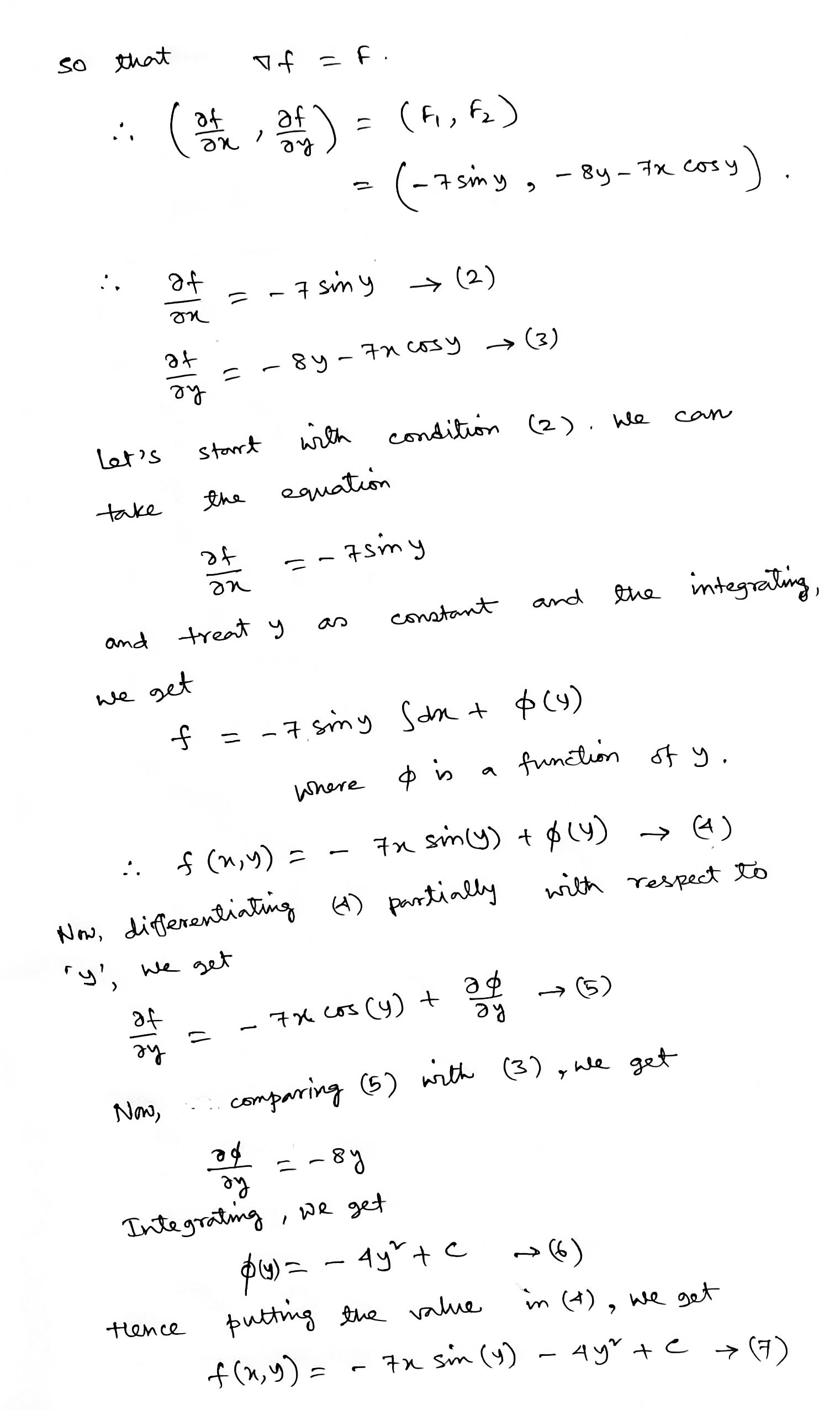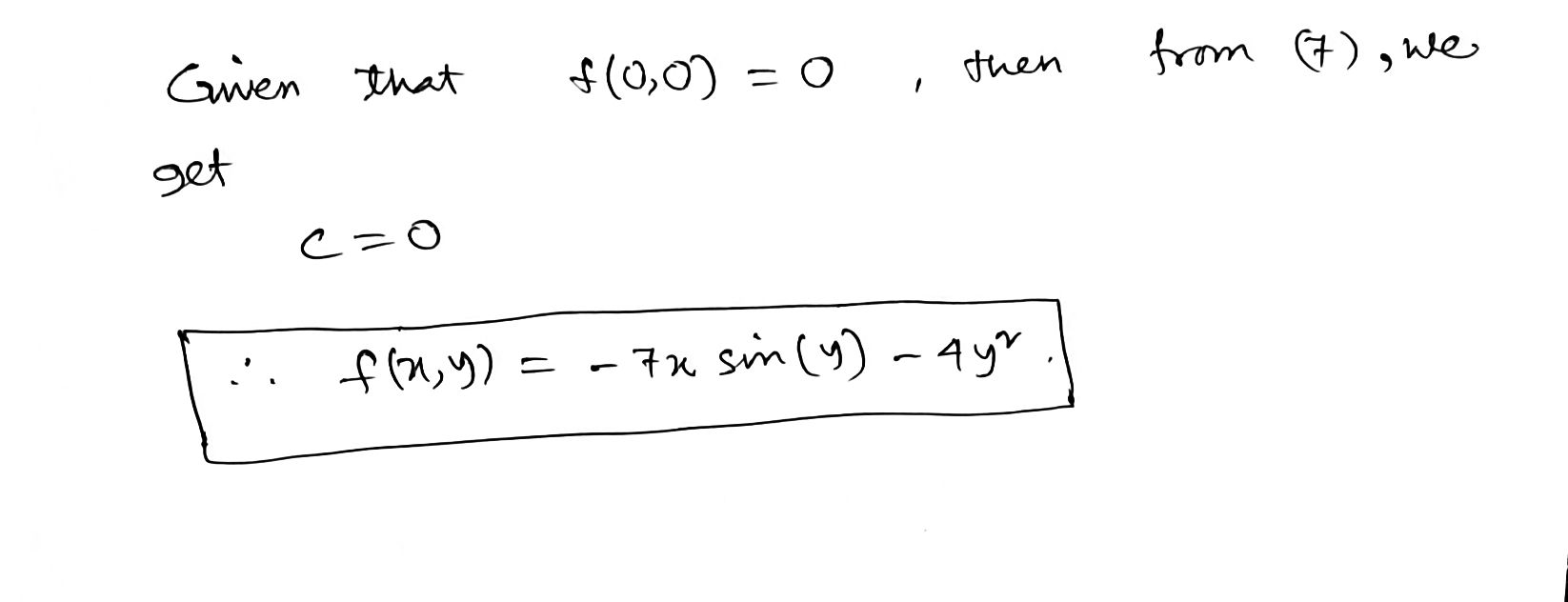Please give a thumbs up if you are benefited.

##### Add Answer of: (1 point) For each of the following vector fields F, decide whether it is conservative or...
Similar Homework Help Questions
• ### (1 point) For each of the following vector fields F decide whether it is conservative or...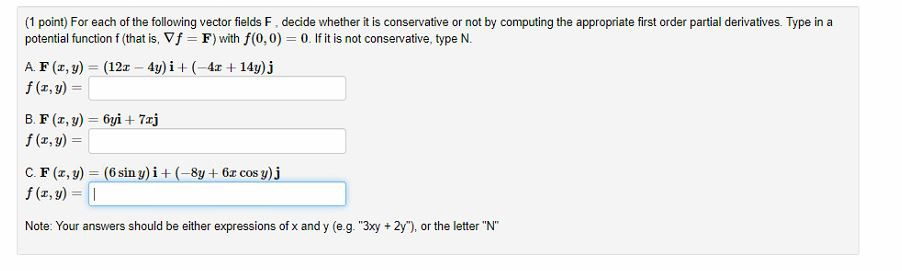(1 point) For each of the following vector fields F decide whether it is conservative or not by computing the appropriate first order partial derivatives. Type in a potential function f (that is, Vf = F) with f(0,0) = 0. If it is not conservative, type N. A. F(, y) = (12x - 4y)i + ( 4x + 14y)j f (1,y) = B.FI,y) = 6yi + 7xj f (, y) = (6 sin y)i + (-8y + 6.0 cos y).j...

• ### (1 point) For each of the following vector fields F, decide whether it is conservative or...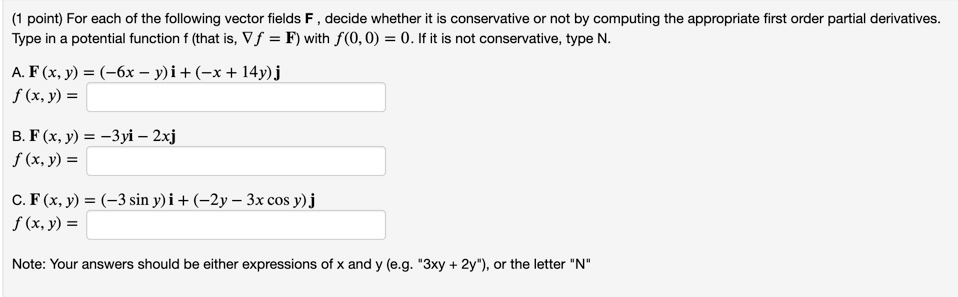(1 point) For each of the following vector fields F, decide whether it is conservative or not by computing the appropriate first order partial derivatives. Type in a potential function f (that is, V f = F) with f(0,0) = 0. If it is not conservative, type N. A. F(x, y) = (-6x - y)i + (-x + 14y)j f (x, y) = B. F(x, y) = -3yi – 2xj f (x, y) = C. F(x, y) = (-3 sin...

• ### For each of the following vector fields F , decide whether it is conservative or not by...

For each of the following vector fields F , decide whether it is conservative or not by computing curl F . Type in a potential function f (that is, gradient f=F). Ifit is not conservative, type N.F(x,y)=(-6x+5y)i+(5x+10y)jF(x,y,z)=-3xi-2yj+kF(x,y)=(-siny)i+(10y-3xcosy)jF(x,y,z)=-3x^2i+5y^2j+5z^2kI don't understand how to calculate any of these. I know that they are all conservative.

• ### decide whether it is conservative or not by computing curl F

For each of the following vector fields F , decide whether it is conservative or not by computing curl F . Type in a potential function f (that is, gradient f=F). Ifit is not conservative, type N.F(x,y)=(-6x+5y)i+(5x+10y)jF(x,y,z)=-3xi-2yj+kF(x,y)=(-siny)i+(10y-3xcosy)jF(x,y,z)=-3x^2i+5y^2j+5z^2kI don't understand how to calculate any of these. I know that they are all conservative.

• ### Question 2 For the following vector fields F determine whether or not they are conservative. For ...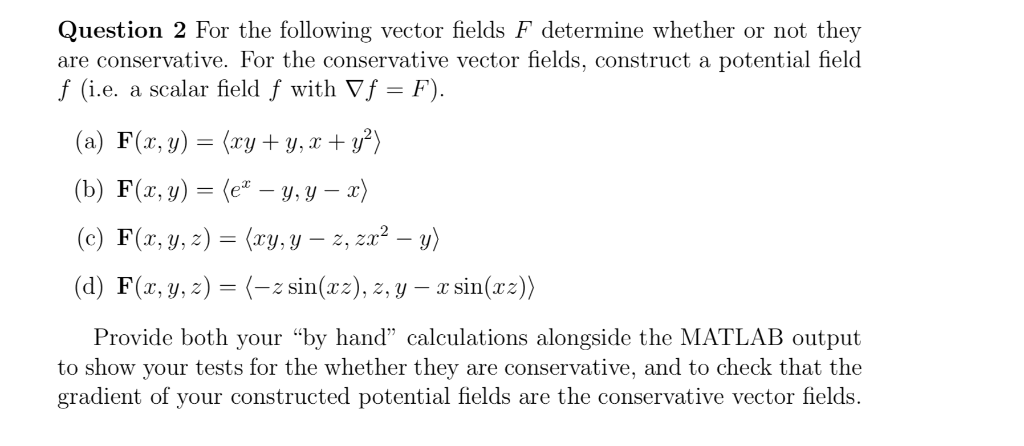Only the Matlab part !!! Question 2 For the following vector fields F determine whether or not they are conservative. For the conservative vector fields, construct a potential field f (i.e. a scalar field f with Vf - F) (a) F(z, y)(ryy,) (b) F(z, y)-(e-y, y-z) (c) F(r, y,z) (ry.y -2, 22-) (d) F(x, y, z)=(-, sin(zz),2, y-rsin(x:) Provide both your "by hand" calculations alongside the MATLAB output to show your tests for the whether they are conservative, and to...

• ### Is Vector fields conservative.

Determine whether or not the given vector fields areconservative.If it is conservative, find the function f suchthat F=delta f1.)F(x,y,z)= (x)i + (y)j + (x)k2.)F(x,y,z)= (yz)i + (y^2 + xz)j + (xy)k

• ### Conservative vector fields

Determine whether the vector field is conservative. If it is find a function f such that F = delta f.F(x,y,z) = xyz^2 i + x^2yz^2 j + x^2y^2z k

• ### 1. (20 points) Identify if the following vector fields are conservative. If there exists a vector...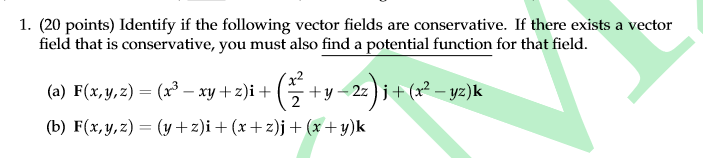1. (20 points) Identify if the following vector fields are conservative. If there exists a vector field that is conservative, you must also find a potential function for that field. (a) F(x,y,z) = (x3 – xy +z)i + 2 (b) F(x,y,z) = (y+z)i + (x+z)j + (x+y)k (& +y +y-22) i + (- y2)k

• ### Calculus #11

For each of the following vector fields F , decide whether it is conservative or not by computing curl F . Type in a potential function f (that is, gradient f=F ). Ifit is not conservative, type N.A) F(x,y) = (4x+3y)i+(3x+6y)jf(x,y) = ?(B) F(x,y) = 2yi+3xjf(x,y) = ?(C) F(x,y) = 2xi+3yj+kf(x,y) = ?(D) F(x,y) = (2siny)i+(6y+2xcos(y))jf(x,y) = ?(E) F(x,y) = 2x^2i+3y^2j+3z^2kf(x,y) = ?

• ### Problem 7. Given that each of the following vector fields F is conservative Find a potential...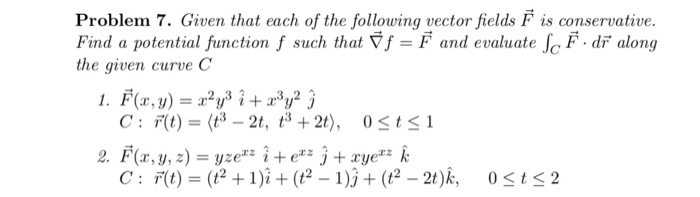Problem 7. Given that each of the following vector fields F is conservative Find a potential function f such that f = F and evaluate fe F dr along the given curve C 1. F(r,y) y C: F(t)(t3- 2t, t3 + 2t), 0 <t<1 2. F(x,y, ) yze"* i + e#* j + xye k C: F(t)(t2 1)i +(2 -1)( -2t)k, 0t 2

Free Homework App